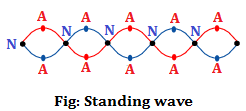# Equation of Stationary Wave

The wave which does not transmit energy from one point to another point of a medium but can distribute energy within the space of the medium is called stationary wave. It is also known as standing wave.

Standing wave is formed when two identical wave travelling from opposite direction superpose to each other. In standing wave nodes and antinodes are formed alternately.

In standing wave, the point in which amplitude become maximum is called antinode and the point in which amplitude becomes zero is called node.Equation of Stationary wave:

Suppose a plane progressive wave travelling form left to right with amplitude a, angular frequency f and wavelength λ. Now the displacement of the particle of a medium is given by:

$$y = aSin (\omega T – kx)…..(ii)$$

Let us consider another identical progressive wave from left t right. Due to this, the displacement of the particle of the medium at that time is given by:

$$y = aSin (\omega T + kx)…..(ii)$$

Since the displacement of the particle is along the same direction, when there wave superpose to each other at any point of the medium. Then using the principle of superposition, the resultant displacement is given by:

$$y = y_1 + y_2$$

$$or, y = aSin (\omega T – kx) + aSin (\omega T + kx)$$

$$or, y = a.(2Sin \omega T -kx) + Sin (\omega T + kx)$$

$$or, y = a.2Sin \frac{\omega T -kx + \omega T + kx}{2} . Cos \frac{\omega T -kx – \omega T – kx}{2}$$

$$\therefore Y = 2acos \frac{2 \pi }{ \lambda } x.sin \omega T$$

This is the required equation.

Do you like this article ? If yes then like otherwise dislike : 3

#### One Response to “Equation of Stationary Wave”

1.